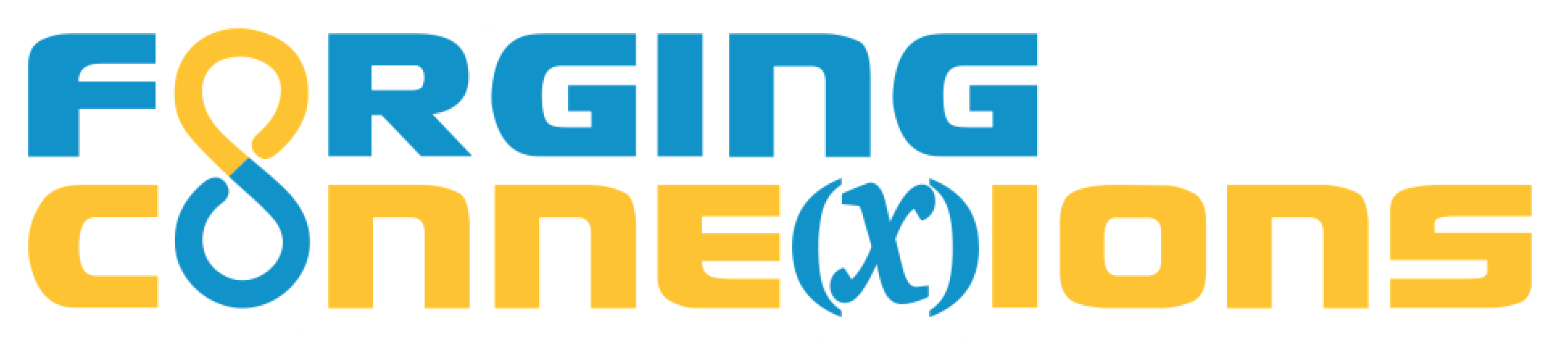This site supports the NSF project on Technology-Rich Units for Future Secondary Teachers: Forging Dynamic Connections Between Geometry and Functions, which is part of the NSF's program on Improving Undergraduate STEM Education (IUSE).

Unit 4: Connect Geometry and Algebra Through Functions

1. Flatland to NumberLand: In this lesson, you’ll restrict the domain of each geometric transformation family (reflect, rotate, translate, glide reflect, dilate) to a number line, and decide which of these families can be arranged to have their range on the same nummber line. For each family, you’ll consider how the numbers corresponding to the independent and dependent variables are related.
2. Construct a Dynagraph: In this lesson, you'll compose two geometric functions, using one number line for the independent variable and putting the composition’s dependent variable on a separate parallel number line. Using parallel lines, and constructing a segment to connect the independent and dependent variables, will make it particularly easy to observe and analyze the relative motion of both variables!
3. Dynagraphs in Algebra: In this lesson, students use dynagraphs without numbers to practice their observation skills, comparing the relative direction and speed of the independent and dependent variables. Students then use dynagraphs with numbers to relate these same speed-and-direction behaviors to functions expressed algebraically and to determine the input or output values designated by expressions using function notation.
4. Construct a Cartesian Graph: In this lesson, you'll compose two geometric functions, using a horizontal number line for the independent variable and using rotation to put the dependent variable on a separate perpendicular number line. Using perpendicular lines will make it particularly easy to a trace a point that shows the relative motion of both variables!